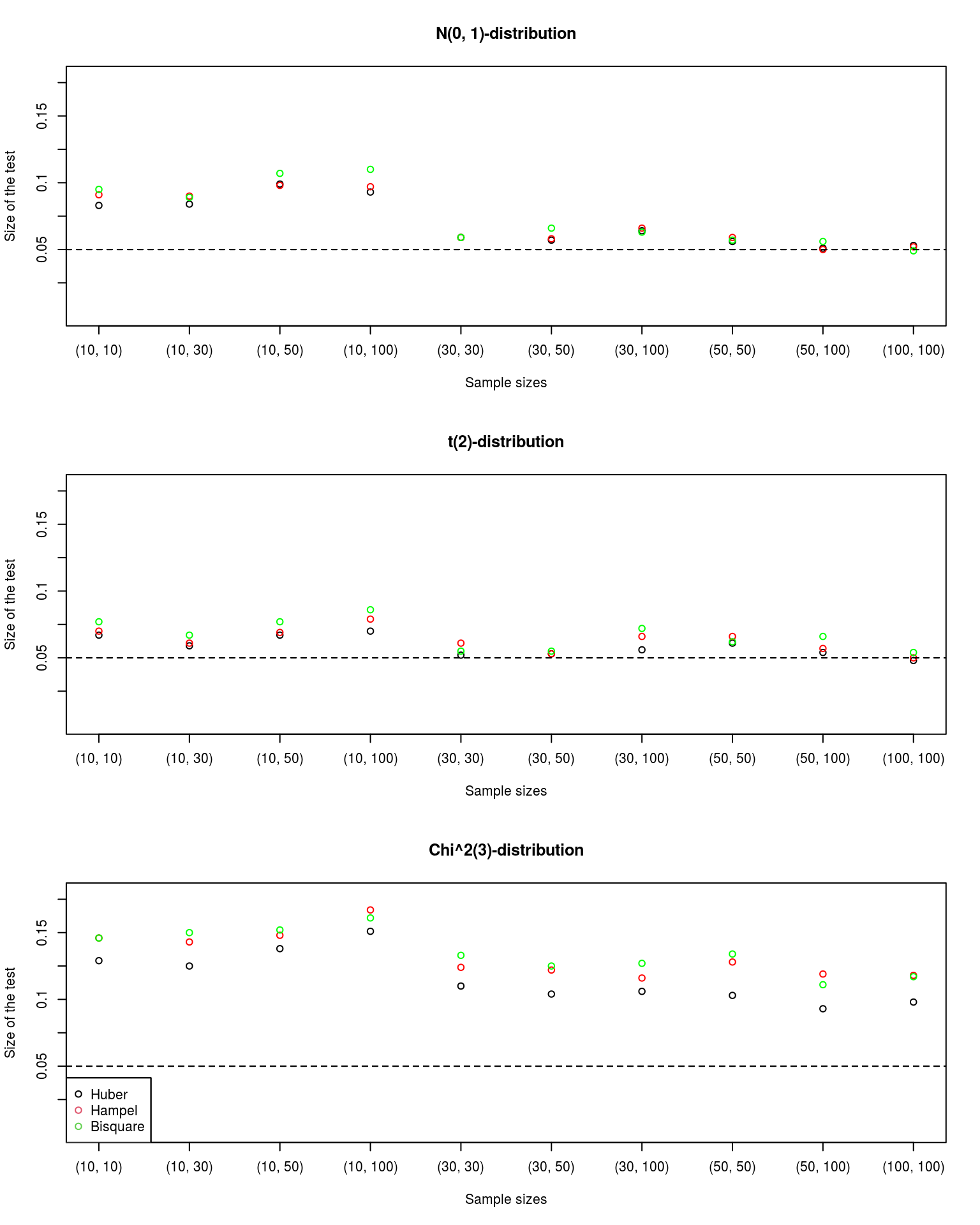# Introduction

In this vignette, we will briefly describe and motivate how we constructed the test statistics used by the function m_test and how it derives a test decision.

# Asymptotic distribution of the M-test statistics

For a more detailed description of the asymptotic behaviour of M-estimators, we refer to Maronna et al. (2006, p. 36ff.), which is the main reference for the following motivation.

We consider two independent samples $$X_1, \ldots, X_m$$ and $$Y_1, \ldots, Y_n$$ of i.i.d. random variables which are symmetrically distributed with variances $$\sigma^2_X$$ and $$\sigma^2_Y$$.

For M-estimators $$\hat{\mu}_X$$ and $$\hat{\mu}_Y$$ with a $$\psi$$-function $$\psi$$, it can be shown under these conditions that \begin{align*} \sqrt{m} \cdot \left(\hat{\mu}_X - \mu_X\right) \overset{\text{asympt.}}{\sim} \mathcal{N}\left(0, \sigma_X^2 \cdot \nu_X\right) \quad \text{and} \quad \sqrt{n} \cdot \left(\hat{\mu}_Y - \mu_Y\right) \overset{\text{asympt.}}{\sim} \mathcal{N}\left(0, \sigma_Y^2 \cdot \nu_Y\right), \end{align*}

where $$\mu_X \in \mathbb{R}$$ and $$\mu_Y \in \mathbb{R}$$ are the values for which \begin{align*} \text{E}\left(\psi\left(\frac{X - \mu_X}{\sigma_X}\right)\right) = 0 \quad \text{and} \quad \text{E}\left(\psi\left(\frac{Y - \mu_Y}{\sigma_Y}\right)\right) = 0, \end{align*}

and

\begin{align*} \nu_X = \frac{\text{E}\left(\psi\left(\frac{X - \mu_X}{\sigma_X}\right)^2\right)}{\left(\text{E}\left(\psi'\left(\frac{X - \mu_X}{\sigma_X}\right)\right)\right)^2} \quad \text{and} \quad \nu_Y = \frac{\text{E}\left(\psi\left(\frac{Y - \mu_Y}{\sigma_Y}\right)^2\right)}{\left(\text{E}\left(\psi'\left(\frac{Y - \mu_Y}{\sigma_Y}\right)\right)\right)^2}. \end{align*}

From this, it follows that \begin{align*} \hat{\mu}_X \overset{\text{asympt.}}{\sim} \mathcal{N}\left(\mu_X, \frac{\sigma^2_X \cdot \nu_X}{m}\right) \quad \text{and} \quad \hat{\mu}_y \overset{\text{asympt.}}{\sim} \mathcal{N}\left(\mu_Y, \frac{\sigma^2_Y \cdot \nu_Y}{n}\right), \end{align*}

implying

\begin{align*} \frac{\hat{\mu}_X - \hat{\mu}_Y - \left(\mu_X - \mu_Y\right)}{\sqrt{\frac{n \cdot \sigma^2_X \cdot \nu_X + m \cdot \sigma^2_Y \cdot \nu_Y}{m \cdot n}}} \overset{\text{asympt.}}{\sim} \mathcal{N}\left(0, 1\right). \end{align*}

In order to use this statistic as a test statistic for our M-tests, we need to estimate $$\sigma_X$$, $$\sigma_Y$$, $$\nu_X$$, and $$\nu_Y$$. We use the $$\tau$$-scale estimator (Maronna and Zamar, 2002) to estimate $$\sigma^2_X$$ and $$\sigma^2_Y$$ by $$\hat{\sigma}_X^2$$ and $$\hat{\sigma}_Y^2$$ robustly and estimate $$\nu_X$$ and $$\nu_Y$$ by

\begin{align*} \hat{\nu}_X = \frac{\frac{1}{m} \sum_{i = 1}^m \psi\left(\frac{X_i - \hat{\mu}_X}{\hat{\sigma}_X}\right)^2}{\left(\frac{1}{m} \sum_{i = 1}^m \psi'\left(\frac{X_i - \hat{\mu}_X}{\hat{\sigma}_X}\right)\right)^2} \quad \text{and} \quad \hat{\nu}_Y = \frac{\frac{1}{n} \sum_{j = 1}^n \psi\left(\frac{Y_j - \hat{\mu}_Y}{\hat{\sigma}_Y}\right)^2}{\left(\frac{1}{n} \sum_{j = 1}^n \psi'\left(\frac{Y_j - \hat{\mu}_Y}{\hat{\sigma}_Y}\right)\right)^2}. \end{align*}

Under the previous considerations, the test statistic of the M-tests we implemented in the package is given by

$\begin{equation*} \frac{\hat{\mu}_X - \hat{\mu}_Y - \Delta}{\sqrt{\frac{n \cdot \hat{\sigma}^2_X \cdot \hat{\nu}_X + m \cdot \hat{\sigma}^2_Y \cdot \hat{\nu}_Y}{m \cdot n}}} \overset{\text{asympt.}}{\sim} \mathcal{N}\left(0, 1\right), \end{equation*}$

where $$\Delta = \mu_X - \mu_Y$$ is the location difference between both distributions.

The M-tests are implemented in the function m_test. More details on the usage of the function can be found in the vignette Getting started with robnptests. Inside m_test, we use the function scaleTau2 from the R package robustbase (Maechler et al., 2020) to compute the $$\tau$$-scale estimates for the samples.

# Simulation results

The following figure shows the simulated test sizes from a small simulation study with 1000 replications, where we applied the M-tests with different $$\psi$$-functions to samples from the $$\mathcal{N}(0, 1)$$-distribution, the $$t_2$$-distribution, and the $$\chi^2_3$$-distribution. We chose the significance level $$\alpha = 0.05$$. The results are shown in the following figure.

Under the $$\mathcal{N}(0, 1)$$- and the $$t_2$$-distribution we make similar observations: For equal sample sizes $$m = n \geq 30$$, the simulated test size is quite close to the the specified value of $$\alpha$$. When $$m \neq n$$, it seems to be important that both values are rather large and do not deviate too much from each other. Otherwise, the tests may become very anti-conservative. In general, the three test statistics lead to similar results for the considered sample sizes.

Under the $$\chi^2_3$$-distribution, all tests are anti-conservative. While there seems to be some improvement when the sample sizes become larger, the estimated sizes are still rather far away from 0.05. A reason might be that the asymptotic variance we use is only a good approximation for symmetric distributions (Maronna et al., 2006, p. 37).Based on these results, we discourage using the tests for asymmetric distributions. For symmetric distributions, the asymptotic test should only be used for large samples. In all other cases, the randomization or permutation test might be preferable.

# Session Info

library(robnptests)

sessionInfo()
#> R version 4.0.3 (2020-10-10)
#> Platform: x86_64-pc-linux-gnu (64-bit)
#> Running under: Linux Mint 19.1
#>
#> Matrix products: default
#> BLAS:   /usr/lib/x86_64-linux-gnu/blas/libblas.so.3.7.1
#> LAPACK: /usr/lib/x86_64-linux-gnu/lapack/liblapack.so.3.7.1
#>
#> locale:
#>   LC_CTYPE=de_DE.UTF-8       LC_NUMERIC=C
#>   LC_TIME=de_DE.UTF-8        LC_COLLATE=C
#>   LC_MONETARY=de_DE.UTF-8    LC_MESSAGES=de_DE.UTF-8
#>   LC_PAPER=de_DE.UTF-8       LC_NAME=C
#>  LC_MEASUREMENT=de_DE.UTF-8 LC_IDENTIFICATION=C
#>
#> attached base packages:
#>  stats     graphics  grDevices utils     datasets  methods   base
#>
#> other attached packages:
#>  robnptests_1.0.0
#>
#> loaded via a namespace (and not attached):
#>   codetools_0.2-16  digest_0.6.27     rbibutils_2.1     R6_2.5.0
#>   jsonlite_1.7.2    magrittr_2.0.1    evaluate_0.14     highr_0.9
#>   Rdpack_2.1.1      rlang_0.4.10      stringi_1.5.3     jquerylib_0.1.3
#>  bslib_0.2.4       rmarkdown_2.7     tools_4.0.3       stringr_1.4.0
#>  xfun_0.22         yaml_2.2.1        compiler_4.0.3    htmltools_0.5.1.1
#>  knitr_1.33        sass_0.3.1